• 二、二叉平衡树调整 三、二叉平衡树的插入 四、二叉平衡树的删除 一、定义 平衡二叉树或则是空树，或者是具有如下特征的二叉排序树 （1）左子树和右子树的深度之差的绝对值不超过1； （2）左子树和右子树也是...
目录

一、定义

二、二叉平衡树调整

三、二叉平衡树的插入

四、二叉平衡树的删除

一、定义

平衡二叉树或则是空树，或者是具有如下特征的二叉排序树

（1）左子树和右子树的深度之差的绝对值不超过1；

（2）左子树和右子树也是平衡二叉树

平衡二叉树的查找的时间复杂度是O(log n)。

某结点的平衡因子：该结点左右子树深度之差。

示例：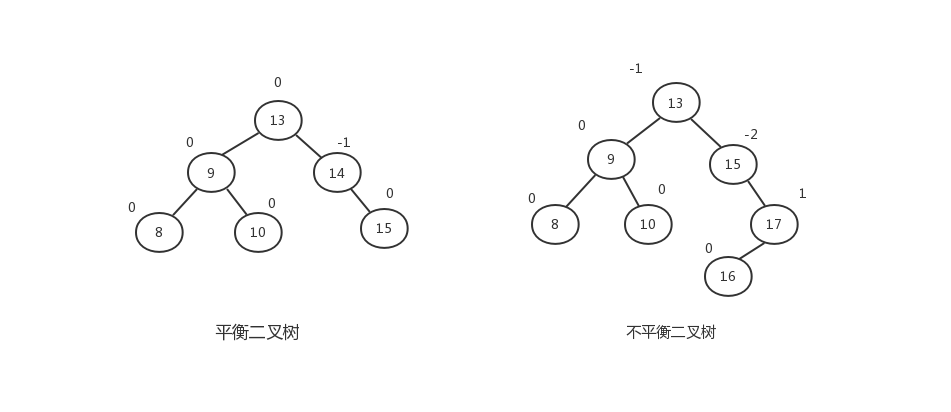二、二叉平衡树调整

在创建平衡二叉树时需要插入结点，插入节点时按照二叉排序树处理，若插入的结点破坏了二叉平衡树的平衡性，则需要对二叉平衡树进行调整。调整的方法是：找到离插入节点最近且平衡因子绝对值超过1的祖先结点，以该结点为根的子树称为最小不平衡二叉树（如上图右边值为15的结点为最小不平衡二叉树的根结点），可将重新平衡的范围局限在这颗子树。

假设最小不平衡二叉树的根结点为A，那么失去平衡后调整的规律可以归纳为以下四种。

（1）LL型：由于在A左子树根节点的左子树上插入节点，使A的平衡因子由1增至2而失去平衡。则需要进行一次向右旋转的操作。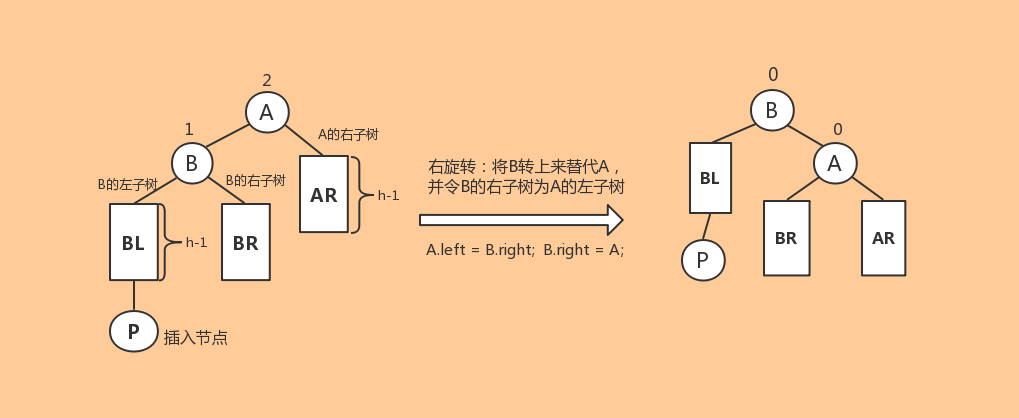两个LL型调整的例子，调整代码都是A.left = B.right; B.right = A：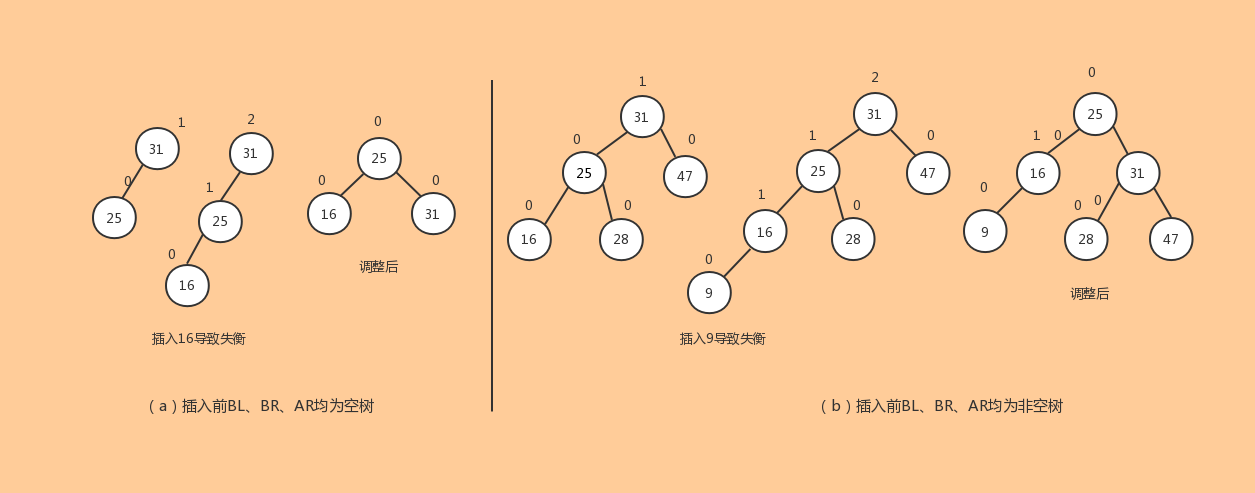（2）RR型：由于在A右子树根节点的右子树上插入节点，A的平衡因子由-1变为-2而失去平衡。则需要进行一次向左旋转的调整。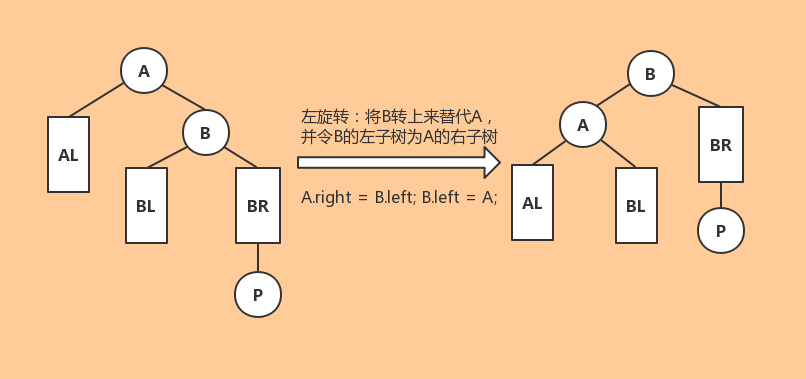RR型调整的例子，调整代码是 A.left = B.right; B.left = A：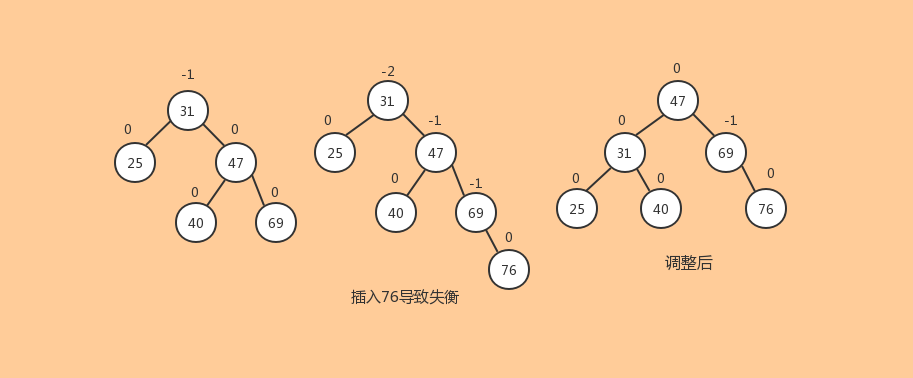（3）LR型：由于在A的左子树根节点的右子树上插入节点，导致A的平衡因子由1增至2，而使A失去平衡，则需要进行两次操作。第一次对B及起右子树进行左旋使之变成LL型，然后对A进行右旋，左旋右旋的代码同上面。（插入结点在C的左子树和在C的右子树上操作相同）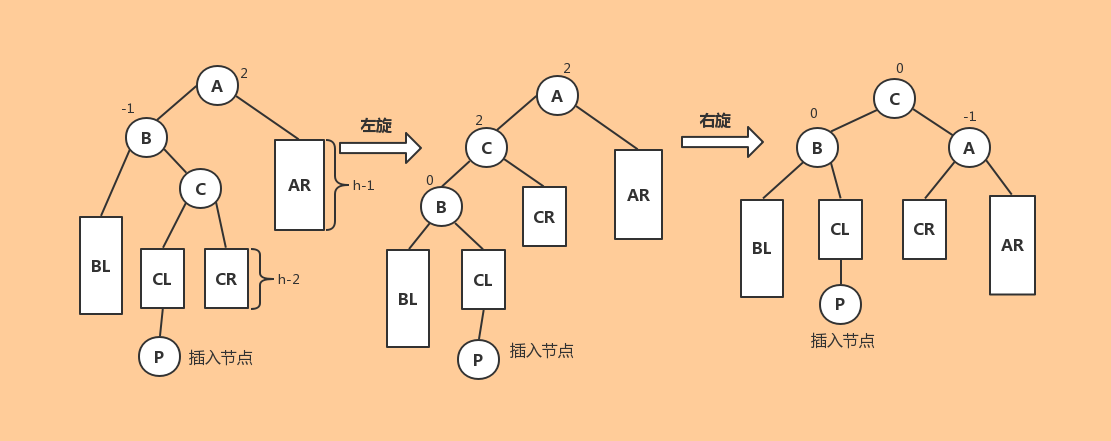LR调整的例子: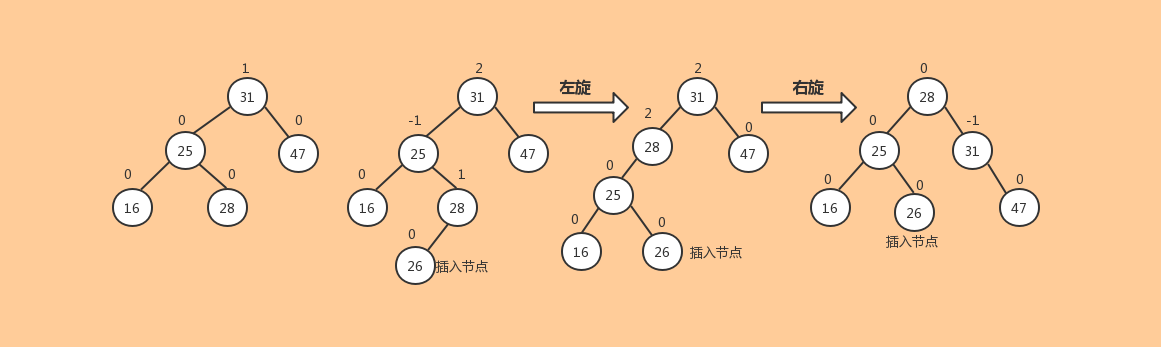（4）RL型：由于在A的右子树根节点的左子树上插入节点，A的平衡因子由-1变成-2，致使以A为根的子树失去平衡，则旋转方法是先对A的右子树右旋，再对A树左旋。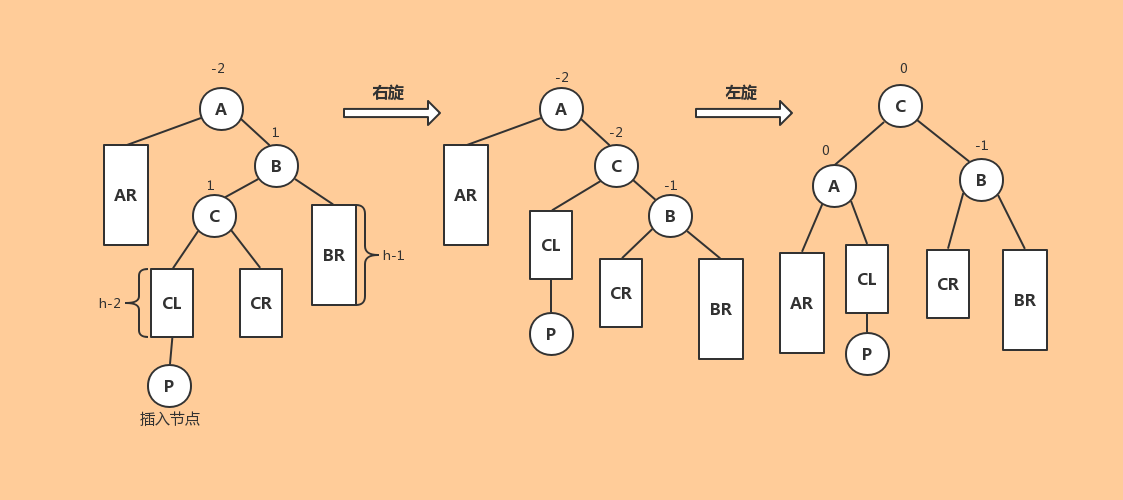RL调整示例：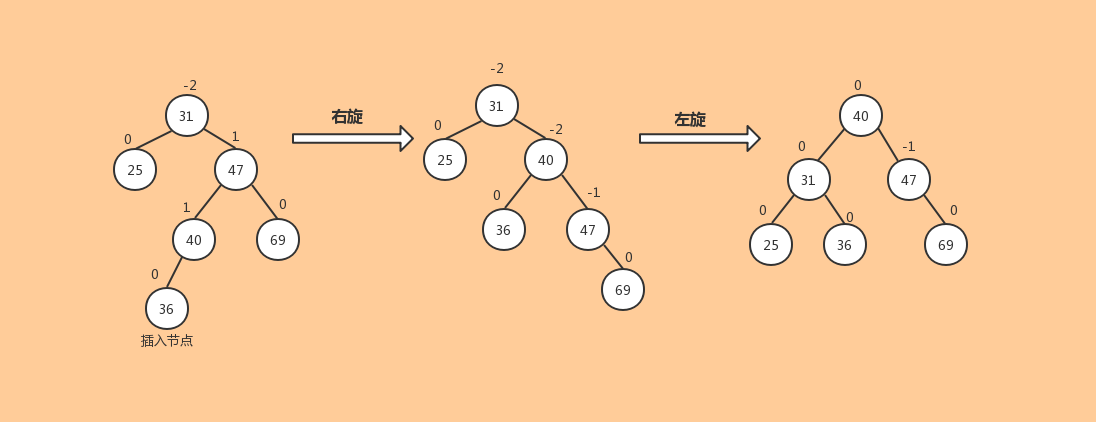调整的实现：

//以p为根的树的右旋转
public AVLNode rightRotation(AVLNode p){
AVLNode b = p.left;		//p的左子树
p.left = b.right;	//旋转后令b的右孩子作为p的左孩子
p.height = Math.max(Height(b.right), Height(p.left))+1; //重新计算p的树深
b.right = p;		//b的右孩子变成p
b.height = Math.max(Height(b.left),Height(b.right))+1; //重新计算b的树深
return b;	//旋转之后b为新的根节点
}

//以p为根的树的左旋转
public AVLNode leftRotation(AVLNode p){
AVLNode b = p.right;	//p的右子树
p.right = b.left;	//旋转后令b的左孩子为p的右孩子
p.height = Math.max(Height(b.left), Height(p.left))+1;	//重新计算p的树深
b.left = p;			//b的左孩子变成p
b.height = Math.max(Height(b.left), Height(b.right))+1;	//重新计算b的树深
return b;	//旋转之后b为新的根节点
}

//先左旋再右旋，先把p的左子树左旋，再对p右旋
private AVLNode LRRotation(AVLNode p){
p.left = leftRotation(p.left);
return rightRotation(p);
}
//先右旋再左旋，先把p的右子树右旋，再对p左旋
private AVLNode RLRotation(AVLNode p){
p.right = rightRotation(p.right);
return leftRotation(p);
}

三、二叉平衡树的插入

通过观察：对于一颗平衡二叉树，假设要插入的结点为P，插入后P的双亲结点为F。

①当插入的结点没有导致子树的深度的增加时，一定不会影响平衡。（F本来有右子树或左子树，P插入后成为F的左子树                 或右子树，树的深度不变，不会影响平衡）

②当插入的结点导致子树的深度增加时：

1.如果影响了平衡，那么经过调整后不会改变最小不平衡二叉树根结点的祖先的平衡因子

2.如果没有影响平衡，那么会影响插入结点点祖先的平衡因子。

③如何确定是否影响了平衡

如果子树的深度增加了，并且某个祖先结点的平衡因子绝对值超过了2，说明影响了平衡。

使用递归来实现插入。递归向下查找时找到插入位置并插入，由于递归在返回时按照查找的路径返回的，所以在返回时碰到的第一个失衡结点就是最小不平衡二叉树的根节点，返回过程中还可以更新结点的平衡因子。为了方便，结点记录以该结点为树根的深度，该结点的平衡因子就是左右子树根节点深度之差。返回时只需要重新计算一次树的深度即可。

结点的数据结构：

static final class AVLNode{
int height;    //以该结点为树根的树的深度
int data;    //值
AVLNode left;    //左孩子
AVLNode right;    //右孩子
AVLNode(){
data = 0;
height =1;
left = right = null;
}
AVLNode(int e){
data = e;
height = 1;
left = right = null;
}
AVLNode(int height,int e,AVLNode left,AVLNode right){
this.height = height;
data = e;
this.left = left;
this.right = right;
}
String toString(){
return data+" ";
}
}

插入算法：

	//获取树深度
private int Height(AVLNode p){
if(p == null)
return 0;
else
return p.height;
}
/*
* 插入操作，递归实现
* 在递归查找时可以找到插入位置，在返回时遇到的第一个不平衡的结点就是最小平衡
* 二叉树的根结点。而且可以根据是在该结点的左子树还是右子树上插入的来判断调整
* 的类型。
*/
private AVLNode insertAVL(AVLNode p,int e){
if(p == null){	//找到插入的位置
p = new AVLNode(e);
}
else if( e < p.data){	//递归查询左子树
p.left = insertAVL(p.left,e);
if(Math.abs(Height(p.left) - Height(p.right))== 2){	//插入后影响平衡
if(e > p.left.data){	//说明是在p的左子树的右子树上插入，为LR型
p = LRRotation(p);
}
else{	//否则为LL型，进行右旋，右旋前根节点为p，右旋后根节点为返回的结点
p = rightRotation(p);
}
}
}
else if(e > p.data){	//递归查询右子树
p.right = insertAVL(p.right,e);
if(Math.abs(Height(p.left) - Height(p.right))==2){//插入后影响了平衡
if(e > p.right.data){ //说明是在p的右子树的右子树上插入的，为RR型
p = leftRotation(p);
}
else{	//否则为RL型
p = RLRotation(p);
}
}
}
p.height = Math.max(Height(p.left), Height(p.right))+1;	//重新计算p的树高
return p;
}

四、二叉平衡树的删除

假设P是要删除的结点，二叉平衡树的删除使用的是二叉排序树删除的方法，用P的直接前驱来替代P，然后将P的直接前驱删除，并从删除位置开始向上查找最小不平衡树的根节点。在左子树上删除一个结点导致失衡，可以看作是在右子树上插入一个结点导致失衡。如果删除的本来就是叶子结点，或者只有左子树或右子树，那么就直接删除P，然后将其左子树或右子树直接接到P的双亲结点，并向上查找最小不平衡树的根节点。

private AVLNode deleteAVL(AVLNode p,int e){
if(p == null)    //不存在值等于e的结点，则什么都不做
return p;
else if( e < p.data){    //查找p的左子树
p.left = deleteAVL(p.left,e);
}
else if( e > p.data){    //查找p的右子树
p.right = deleteAVL(p.right,e);
}
else{    //找到了值等于e的结点
if( p.left != null && p.right != null){    //如果p有左右子树
AVLNode s = p.left;
while( s.right != null){    //找p的前驱，即左子树最右下的结点
s = s.right;
}
p.data = s.data;    //使用前驱替代p
p.left = deleteAVL( p.left , s.data)    //要删除的结点就成了p的前驱
}
else{    //如果p只有左子树或右子树或都没有
//当要删除的是p的前驱时，最后也是在这删除的，因为p的前驱一定没有右子树

p = p.left == null ? p.right : p.left;    //将要删除结点的非空子树挂在其双亲结点
}
}
//判断是否影响了平衡并调整树
if( p == null)
return p;
else if(Height(p.left) - Height(p.right) >=2){    //由于左子树比右子树高影响平衡
if( Height(p.left.left) > Height(p.left.right) ){    //LL型
p = leftRotation(p);
}
else{    //LR型
p = LRRotation(p);
}
}
else if(Height(p.right) - Height(p.left) >=2){    //由于右子树比左子树高影响平衡
if(Height(p.left.left) > Height(p.left.right)){    //RL型
p = RLRotation(p);
}
else{    //RR型
p = rightRotation(p);
}
}
p.Hieght = Math.max(Height(p.left),Height(p.right)) + 1;    //重新计算p的深度
return p;
}



完整代码：

package trees;
import java.util.*;
//二叉平衡树（AVL树）
public class AVLTree {
static final class AVLNode{
int height;	//以该结点为根的树的深度，平衡因子为左右子树深度之差
int data;	//值
AVLNode left;	//左孩子
AVLNode right;  //右孩子
AVLNode(){
height = 1;
data = 0;
left = right = null;
}
AVLNode(int height,int e,AVLNode left,AVLNode right){
this.height = height;
this.data = e;
this.left = left;
this.right = right;
}
AVLNode(int e){
data = e;
height = 1;
left = right = null;
}
public String toString(){
return data+" ";
}
}
HashMap<String,String> s = new  HashMap<>();

AVLNode root;
public AVLTree(){
root = null;
}
//获取树深度
private int Height(AVLNode p){

if(p == null)
return 0;
else
return p.height;
}

//以p为根的树的右旋转
public AVLNode rightRotation(AVLNode p){
AVLNode b = p.left;		//p的左子树
p.left = b.right;	//旋转后令b的右孩子作为p的左孩子
p.height = Math.max(Height(b.right), Height(p.left))+1; //重新计算p的树深
b.right = p;		//b的右孩子变成p
b.height = Math.max(Height(b.left),Height(b.right))+1; //重新计算b的树深
return b;	//旋转之后b为新的根节点
}

//以p为根的树的左旋转
public AVLNode leftRotation(AVLNode p){
AVLNode b = p.right;	//p的右子树
p.right = b.left;	//旋转后令b的左孩子为p的右孩子
p.height = Math.max(Height(b.left), Height(p.left))+1;	//重新计算p的树深
b.left = p;			//b的左孩子变成p
b.height = Math.max(Height(b.left), Height(b.right))+1;	//重新计算b的树深
return b;	//旋转之后b为新的根节点
}

//先左旋再右旋，先把p的左子树左旋，再对p右旋
private AVLNode LRRotation(AVLNode p){
p.left = leftRotation(p.left);
return rightRotation(p);
}
//先右旋再左旋，先把p的右子树右旋，再对p左旋
private AVLNode RLRotation(AVLNode p){
p.right = rightRotation(p.right);
return leftRotation(p);
}
/*
* 插入操作，递归实现
* 在递归查找时可以找到插入位置，在返回时遇到的第一个不平衡的结点就是最小平衡
* 二叉树的根结点。而且可以根据是在该结点的左子树还是右子树上插入的来判断调整
* 的类型。
* 如果插入导致失衡，假设A为失衡后的最小不平衡二叉树，经过旋转调整后树深度其实
* 不会改变，但是A的子树的树深度可能由于调整变化了位置，树深度可能变化。
* 只有在插入后树没有失衡时才可能导致树的深度增加。
*/
private AVLNode insertAVL(AVLNode p,int e){
if(p == null){	//找到插入的位置
p = new AVLNode(e);
}
else if( e < p.data){	//递归查询左子树
p.left = insertAVL(p.left,e);
if(Math.abs(Height(p.left) - Height(p.right))== 2){	//插入后影响平衡
if(e > p.left.data){	//说明是在p的左子树的右子树上插入，为LR型
p = LRRotation(p);
}
else{	//否则为LL型，进行右旋，右旋前根节点为p，右旋后根节点为返回的结点
p = rightRotation(p);
}
}
}
else if(e > p.data){	//递归查询右子树
p.right = insertAVL(p.right,e);
if(Math.abs(Height(p.left) - Height(p.right))==2){//插入后影响了平衡
if(e > p.right.data){ //说明是在p的右子树的右子树上插入的，为RR型
p = leftRotation(p);
}
else{	//否则为RL型
p = RLRotation(p);
}
}
}
p.height = Math.max(Height(p.left), Height(p.right))+1;	//重新计算p的树高
return p;
}
/*
* 删除操作
* 由二叉排序树的删除可知，假设要删除的结点为p，删除一般使用前驱后者后继来替代要删除的结点，
* 然后再删除掉前驱或者后继。二叉平衡树的删除也类似处理，在删除后要调整树
*/
private AVLNode deleteAVL(AVLNode p,int e){
if( p == null)	//不存在值等于e的结点
return p;
else if( e < p.data){
p.left = deleteAVL(p.left,e);	//在左子树中删除
}
else if( e > p.data ){
p.right = deleteAVL(p.right,e);	//在右子树中删除
}
else{	//找到了值等于e的结点
//如果既有左子树又有右子树，那么使用前驱替代的方法
if( p.left != null && p.right !=null ){
AVLNode s = p.left;
while(s.right != null){
s = s.right;
}
p.data = s.data;
//删除前驱，即删除p的左子树中值等于s.data的结点
p.left = deleteAVL(p.left,s.data);
}
else{
/*
* 如果不是左右子树都为空，那么只需要用p的左右子树替代p即可
* 最后删除结点的语句就是这一句。。。
*/
p = p.left == null? p.right:p.left;
}
}

//调整平衡，在p的右子树上删除一个结点导致失衡可以看做是在p的左子树上插入一个结点导致失衡
if( p == null){
return p;
}
if(Height(p.left) - Height(p.right) >= 2){	//由于左子树高而失衡
//相当于在左子树的左子树上插入一个结点导致失衡,LL型
if(Height(p.left.left)>Height(p.left.right)){
return rightRotation(p);
}
else{	//LR型
return LRRotation(p);
}
}
else if(Height(p.right) - Height(p.left) >= 2){ //由于右子树高而失衡
if(Height(p.right.right) > Height(p.right.left)){ //RR型
return rightRotation(p);
}
else{	//RL型
return RLRotation(p);
}
}
p.height = Math.max(Height(p.left), Height(p.right))+1;	//调整树高
return p;
}
public void inOrder(){
inOrder(root);
}
public void inOrder(AVLNode p){
if(p != null){
inOrder(p.left);
System.out.print(p+" ");
inOrder(p.right);
}
}
public void insertAVL(int e){
root = insertAVL(root,e);
}
public void deleteAVL(int e){
root = deleteAVL(root,e);
}
public static void main(String[] args) {
// TODO Auto-generated method stub
Random rand = new Random();
AVLTree t = new AVLTree();
for(int i = 0;i < 10;i++){
t.insertAVL(rand.nextInt(100)+1);
}
t.inOrder();
System.out.println();
int a;
Scanner scan = new Scanner(System.in);
a = scan.nextInt();
t.deleteAVL(a);
t.inOrder();
}
}


几乎完全参考自：https://blog.csdn.net/shichimiyasatone/article/details/85231211

展开全文AVL树
• 二叉查找树的缺点 ...二叉平衡树是一颗要求左右子树的高度差不能超过1的二叉查找树，这个要求使得每次在进行插入、删除的时候，这棵树都可能遭到破坏，进而需要进行左旋右旋等操作恢复平衡。当我们...
二叉查找树的缺点
一般的二叉查找树，在查找某个节点值时，可采用类似二分法的思想进行查找，时间复杂度为O(log（n）)；但是当二叉查找树在极端情况下退化为类似于链表时，时间复杂度为O(n)；
这时二叉平衡树应运而生；
二叉平衡树的缺点
二叉平衡树是一颗要求左右子树的高度差不能超过1的二叉查找树，这个要求使得每次在进行插入、删除的时候，这棵树都可能遭到破坏，进而需要进行左旋右旋等操作恢复平衡。当我们的插入删除操作特别频繁时，会使得操作效率非常低下；
这时红黑树的优点就显现出来了；
红黑树的优点
红黑树也是一颗查找树，他要求：
1：根节点是黑色的；
2：每隔叶子结点都是黑色的空节点；
3：任何相邻的节点都不能同时为红色；
4：每个节点，其到达叶子结点的所有路径中都拥有相同数量的黑色节点。
这些特性使得红黑树可以在哪怕最坏情况下也能在O（log（n））时间复杂度找到特定节点。


展开全文数据结构
• 二叉平衡树就是在二叉搜索树的基础上进行了 优化 二叉平衡树： 空二叉搜索树或者根的左右子树 高度之差绝对值 不超过1；左、右均是 二叉平衡树 二叉树结点的平衡因子：结点的左右子树的高度差 感觉二叉 平衡树...
二叉平衡树就是在二叉搜索树的基础上进行了 优化

二叉平衡树： 空二叉搜索树或者根的左右子树 高度之差绝对值 不超过1；左、右均是 二叉平衡树

二叉树结点的平衡因子：结点的左右子树的高度差

感觉二叉 平衡树主要内容就在于平衡调整上面

在我们插入新的元素后可能会导致原来的二叉平衡树不再平衡，此时我们只需从新插入结点到根节点的方向找到最小二叉平衡树 将其调整为 平衡即可。

可以分为单一型和混合型。

单一 型：LL或RR

混合型：LR或RL

从最小不平衡的子树的根节点（平衡因子绝对值大于1）到新插入节点方向，将路过的三个结点依次记为s,r,u

上面比如LL的意思：新元素插在了s的左孩子的左子树上。

根据 单一型或者混合型 可以分为两种 调整方式，单旋转和双旋转。单一型的用 单旋转，混合型的 用双旋转。

单旋转：s,r,u中，r篡位成为r原先的父节点

双旋转：s,r,u中，u篡位成为u原先的父节点

下面是三个例子：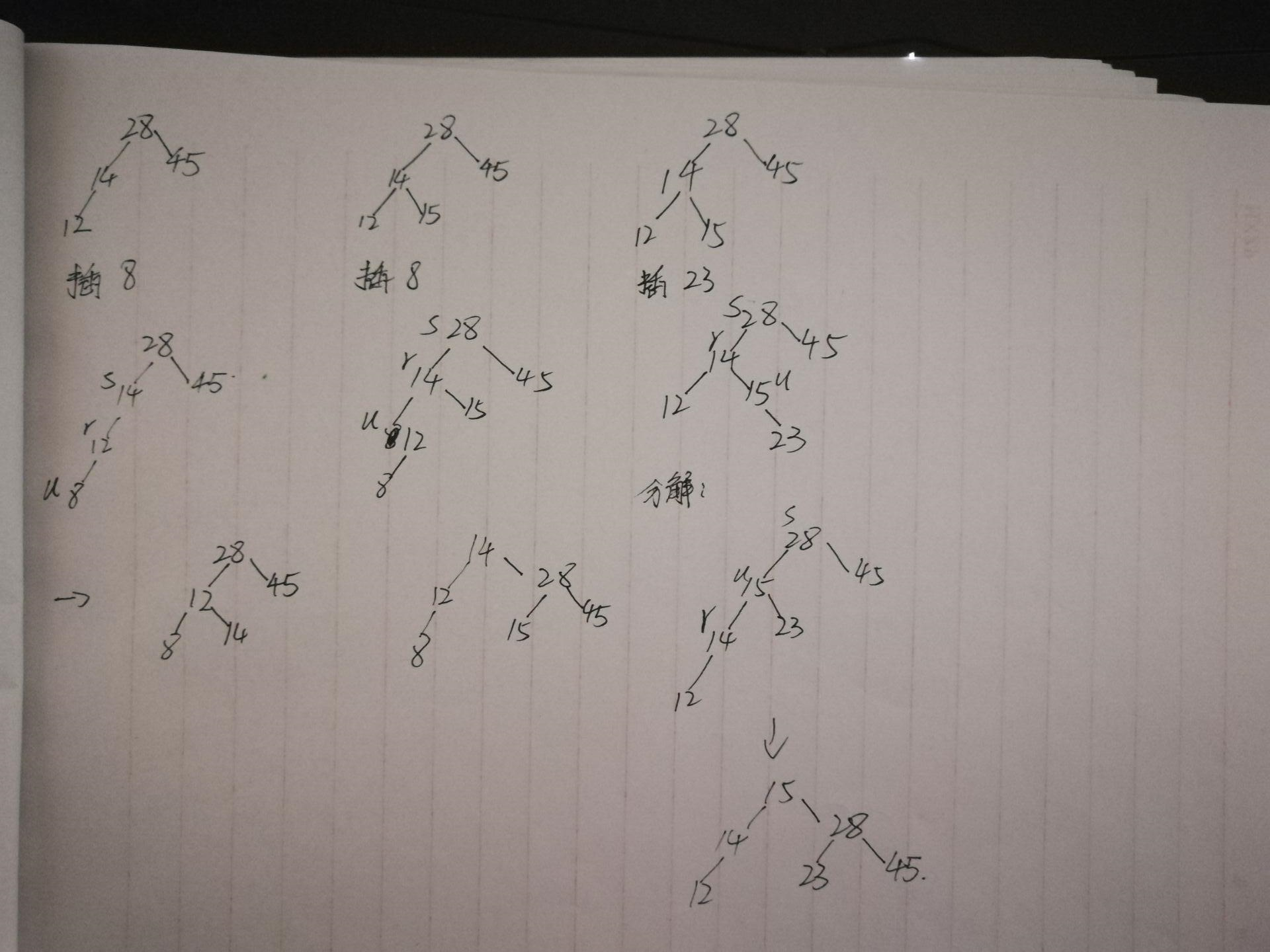展开全文数据结构
• 二叉平衡树： 特性： 1.左子树与右子树的深度差，最大不能超过1. 2.二叉平衡树是二叉排序演变 以上查找一个数据的时间复杂度最大为 O(logn) 红黑树 就是 二叉平衡树 B树： 是多叉二叉平衡树。 特性：...
二叉排序树

特性

1.左节点数据 < 根节点数据 < 右节点数据

2. 每个节点的度最大为2

二叉平衡树：

特性：

1.左子树与右子树的深度差，最大不能超过1.

2.二叉平衡树是二叉排序演变

以上查找一个数据的时间复杂度最大为 O(logn)

红黑树 就是 二叉平衡树B树： 是多叉二叉平衡树。

特性： 1、控制树的高度   2、横向扩展

特点： 每层元素从左到有逐渐递增。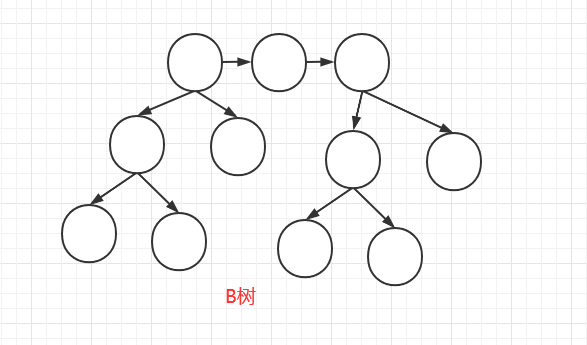B树由红黑树演变而来

为什么会有这种演变呢？

假设有10W条数据，树的深度h将会很大，查询时间复杂度 O(log h), h越大，所花费的时间越高。. 如果查询的是多个数据，那么时间复杂度将是 O(m log h)。 因此在控制高度的情况下，对红黑树进行横向扩展，就成了B树。

B树时间复杂度:  O（m n / (2^h -1)）  这里只能大致说出 横向时间复杂度 比 纵向要低，而且随着数量的越多，优势越明显。

B+树，由B树演变而来，用于索引。

特性：

1.非叶子节点，存储的是指针。 叶子节点，存储的是数据集。

2.叶子节点有指针指向，便于进行范围式查找。 (优点)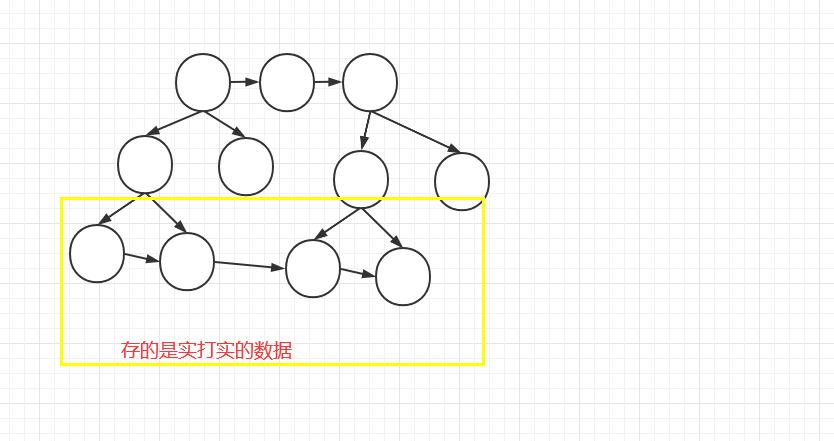展开全文• 二叉搜索树、二叉平衡树、红黑树的区别与应用场景。
• 因为二叉搜索树有可能因为插入的节点大部分都大于、或者大部分都小于根节点，使得二叉树节点增加严重向左/右某一边倾斜，从而导致搜索性能大打折扣，所以引入了二叉平衡树。跟二叉搜索树相比，它的特点是可以通过...算法 数据结构
• 【说明】：博客内容选自课程课件 已知长度为12的表：  (Jan,Feb,Mar,Apr,May,Jun,Jul,Aug,Sep,Oct,Nov,Dec) ...2.按表中元素的顺序依次插入生成一颗二叉排序(初始为空)，并求其在等概率的情况下...
• 动态查找主要包括：二叉查找平衡二叉树，红黑，B，B-，查找的时间复杂度就为O(log2N)，通过对数就可以发现降低的深度就会提高查找效率 需要的应用场景 大量的数据会存储到外存磁盘，外存磁盘中...
• ## 创建二叉平衡树

千次阅读 2016-08-10 15:45:38
那么如何根据升序数组建立二叉平衡树，很简单，只需找到中间节点作为根，同理递归建立左右子树即可。 代码 /* 题目描述 对于一个元素各不相同且按升序排列的有序序列，请编写一个算法，创建一棵高度最小的二叉...
• 这时候二叉平衡树应运而生。 二叉平衡树就是任何一个节点的左右子树的深度之差不能超过一； 既然要用到深度，那就应该有一个计算给定节点左右子树深度的函数，而计算左右子树深度都相当于计算指定节点的深度，而一个...算法
• 二叉排序平衡二叉树，红黑总结 二叉排序 二叉排序，相信大家都接触过，二叉查找的特点就是左子树的节点值比父亲节点小，而右子的节点值比父亲节点大，如图 基于二叉查找的这种特点，我们在查找某个...算法 数据结构 java
• ## 二叉平衡树的旋转操作

万次阅读 多人点赞 2018-06-25 00:33:21
旋转是很多二叉平衡树维持平衡的主要手段，在这里复习一下。其实旋转过程中节点位置的变化只要遵循一个原则就行了：比Root小的在左子树，比Root大的在右子树。（当然这里前提条件是左小右大）。 情况一：插入F节点...数据结构 算法
• 文章目录二叉平衡树的意义二叉平衡树的定义二叉平衡树的作用二叉平衡树的操作平衡因子 二叉平衡树的意义 二叉平衡树的定义 它是一 棵空树或它的左右两个子树的高度差的绝对值不超过1，并且左右两个子树都是一棵平衡...
• 近期在刷树的专题，因而对于树的一些知识进行总结。 如果网上没有较好的，我会自己总结，但是如果有比较好的，我也就没总结的必要了0.0。但总之，希望大家能够更好的学习。 二叉搜索树： ...二叉平衡树： ...
• 【转载】二叉平衡树的插入和删除操作 1.  二叉平衡树 二叉排序树查找、插入和删除操作的时间复杂度和树的深度n有关。构建树时，当先后插入的结点按关键字有序时，二叉排序树退化为单枝树，平均查找长度为(n+1)...
•c++
• AVL二叉平衡树删除--标准版AVL二叉平衡树删除--标准版AVL二叉平衡树删除--标准版AVL二叉平衡树删除--标准版
• 根据二叉平衡树的性质，先判断递归判断左右子树是否为二叉平衡树，随后判断左右子树的高度差是否大于2 2.实现代码 int IsBalance(BiTree T){ //空树为二叉平衡树 if(T==NULL) return 1; //递归判断左右子树是否...
• 首先二叉平衡树依然是一课二叉搜索树，关于二叉搜索树以及其平均查找时间的分析，可以见关于二叉查找树的平均查找时间的问题这一篇。我可能写得不太好，所以最好还是参考一些教材，教材的描述通常会更为严谨，博客...数据结构 Java 二叉搜索树
• 1.二叉排序(BST) 二叉排序是一个递归的数据结构；对二叉树的中序遍历结果为顺序小到大序列； 二叉排序的目的不是为了排序，而是为了提高查找(有序）、和删除关键字（树型结构）的速度； 特点：左子树<根...二叉排序树
• （一）二叉搜索（BST）： 如果一个二叉树满足：对于任意一个节点，其值不小于左子树的任何节点，且不大于右子的任何节点（反之亦可），则为二叉搜索。如果按照中序遍历，其遍历结果是一个有序序列。因此，...
• C语言 二叉平衡树实现学生管理系统，用文件保存学生信息，可以实现学生信息的显示、查找、插入、删除、保存等。
• 前面已经实现了一个二叉搜索树 比较复杂的就是在删除的时候，需要做比较多的判断，总体没那么难理解。...在二叉树的基础上，有一个叫做二叉平衡树的数据结构，实现起来难度还是比较大的。具体代码可参考：AVLTree ...数据结构...